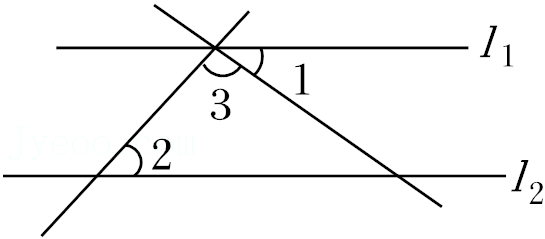$\text{A.}$ $46^{\circ}$ $\text{B.}$ $90^{\circ}$ $\text{C.}$ $96^{\circ}$ $\text{D.}$ $134^{\circ}$
【答案】 C